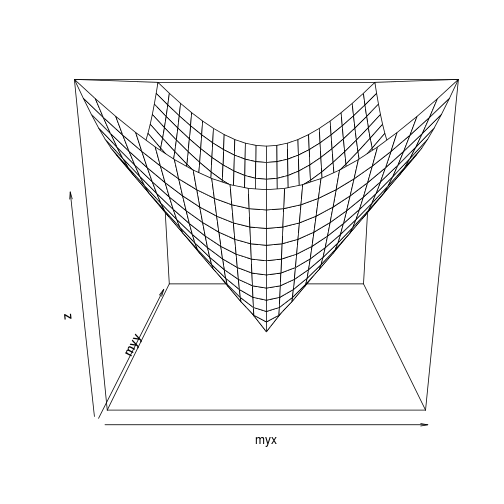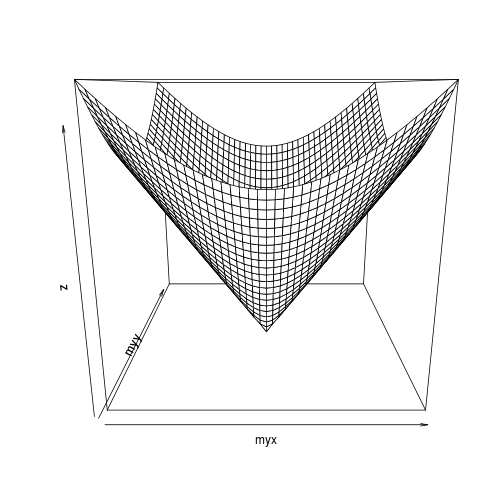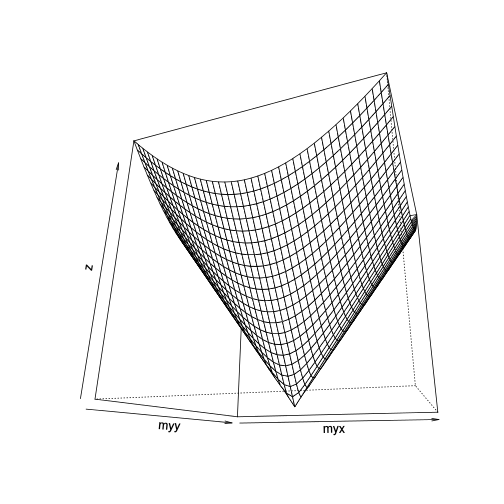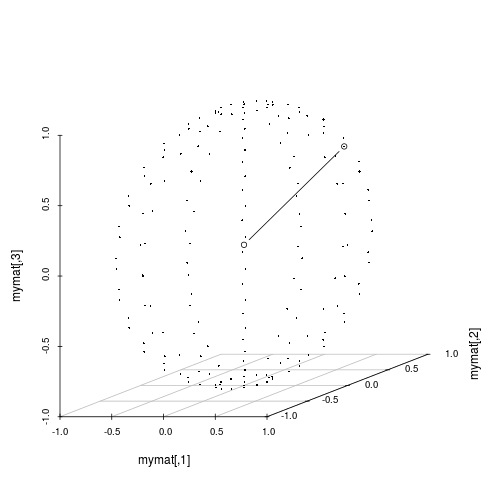## Title: plot-3d-various-1
## Author: Paul Johnson <pauljohn at ku.edu>
## Date posted: 2011->
## Description. A little file of test cases

myx <- seq(-10, 10, by = 1)
myy <- seq(-10, 10, by = 1)
myNorm <- function(x,y) sqrt(x^2 + y^2)
z <- outer(X = myx,Y = myy, function(X,Y) myNorm(X,Y))

persp(myx, myy, z)myx <- seq(-.2, .2, by = 0.01)
myy <- seq(-.2, .2, by = 0.01)
mycircle <- function(x,y) sqrt(x^2 + y^2)
z <- outer(X = myx,Y = myy, function(X,Y) myNorm(X,Y))

persp(myx, myy, z)persp(myx, myy, z, phi = -20, theta = 20)library("rgl")

theta1 <- adf$theta1 theta2 <- adf$theta2
r <- adf$r x0 <- adf$x0
y0 <- adf$y0 z0 <- adf$z0
x <- x0 + r*sin(theta1)*cos(theta2)
y <- y0 + r*sin(theta1)*sin(theta2)
z <- z0 + r*cos(theta1)
cbind(x,y,z)
}

theta1 <- seq(0, pi, length.out=15)
theta2 <- seq(0, 2*pi, length.out=15)
mygrid <- expand.grid(theta1=theta1,theta2=theta2,r=1,x0=0,y0=0,z0=0)

mycoords <- mycirc(mygrid)

mymat <- matrix(mycoords, ncol=3)

plot3d(mymat, col="pink", cex=0.3)

## Warning: font family "sans" not found, using "bitmap"

### add a line
plot3d(matrix(c(0,0,0,mymat[20,]),byrow=T,ncol=3), type="l", add = TRUE)

library(scatterplot3d)

s3d <- scatterplot3d(mymat, pch = 18, cex.s = 0.4, angle = 20, box = FALSE)
newline <- matrix(c(0,0,0,mymat[20,]),ncol=3,byrow=T)
s3d\$points3d (newline,type="b")

## Want to see more? try this
## example(scatterplot3d)

## curve3d is in emdbook
## install.packages("emdbook")
library(emdbook)

## Loading required package: MASS## Please RUN. there are some nice plots they have there
## example(curve3d)

## At one time, I could make this draw in 3d, but fail now
## curve3d( sqrt(x^2 + y^2), from = c(0,0), to = c(0.5,0.5), type = "rgl", add=T)

## http://en.wikipedia.org/wiki/Cone_%28geometry%29

## A right circular cone with height h  and aperture 2θ, whose axis is the z coordinate axis and whose apex is the origin, is described parametrically as

##     S(s,t,u) = \left(u \tan s \cos t, u \tan s \sin t, u \right)

## where s,t,u range over [0,θ), [0,2π), and [0,h], respectively.

## In implicit form, the same solid is defined by the inequalities

##     \{ S(x,y,z) \leq 0, z\geq 0, z\leq h\},

## where

##     S(x,y,z) = (x^2 + y^2)(\cos\theta)^2 - z^2 (\sin \theta)^2.\,

## More generally, a right circular cone with vertex at the origin, axis parallel to the vector d, and aperture 2θ, is given by the implicit vector equation S(u) = 0 where

##     S(u) = (u \cdot d)^2 - (d \cdot d) (u \cdot u) (\cos \theta)^2
## or
##     S(u) = u \cdot d - |d| |u| \cos \theta

## where u = (x,y,z), and u \cdot d denotes the dot product.

## d vector
## aperture 2*theta

## S(u) = 0 = (u * d)^2 - (d * d ) (u * u) (cos(theta))^2

## S(u) = 0 = u * d - |d| |u| cos(theta)

##  x*d1 + y*d2 + z*d3 - |d| sqrt(x^2 + y^2 + z^2) cos(theta)